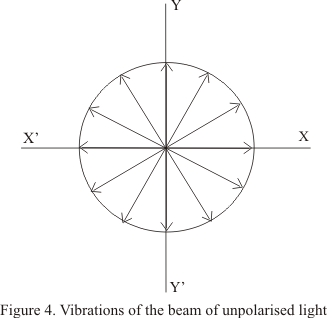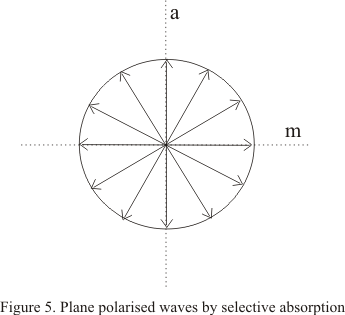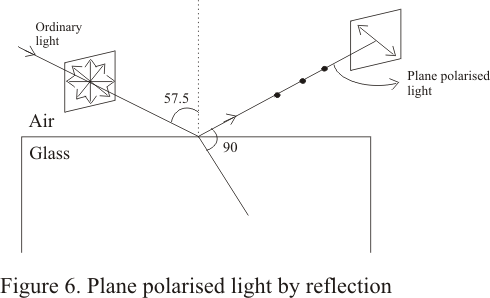# Diffraction and polarization of light

## 5) Vibrations in unpolarized and polarized light

• A light wave is a transverse wave having vibration at right angles to the direction of propagation

• An ordinary beam of light consists of million of lights waves each with its own plane of vibration so it have wave vibrating in all possible plane with equal probability .Hence an ordinary beam of light in unpolarized

• If we consider the light beam being propagated in a direction perpendicular to the plane of paper while its vibrations are in the plane of the paper then figure 4 given below shows that vibrations in ordinary lights occurs in every plane perpendicular to the direction of propagation of light and are in the plane of the paper• As it can be seen from the figure that amplitude of vibrations are all equal

• When such a beam of unpolaroized light is incident on a Polaroid the emergent light is linearly polarized with vibrations in a particular directions

• The direction of vibrations of beam transmitted by the Polaroid depends on the orientation of the Polaroid

• Consider the vibrations in ordinary light when it is incident on the polaroid as shown in figure 3(a). Each vibrations can be resolved into two components, one in a direction parallel to a which is the direction of transmission of light through polaroid and the other direction m perpandicular to a as shown in the figure given below.• Polaroids absorbs the light due to vibrations parallel to m , known as ordinary rays but allow light due to other vibration , known as extra ordinary rays, to pass through.

• So the plane polarised light due to vibrations in one plane is produced as shown in figure 3(a).

• The Polaroid absorbs the light vibration along a particular direction and the component at right angles to it is allowed to pass through the Polaroid.

• This selective absorption of light vibrations along a particular direction is also shown by certain natural crystals for example tourmaline crystal

### 6) Polarization of reflection

• This simple method of obtaining plane polarized light by reflection was discovered by malus in 1808

• We found that when a beam of light is reflected from the surface of a transparent medium like glass or water, the reflected light is partially polarized and degree of the polarization varies with angle of incidence

• The percentage of polarized light is greatest in reflected beam when light beam is incident on the transparent medium with an incident angle equal to the angle of polarization

• For ordinary glass with refractive index =1.52 ,angle of polarization is 57.50

• Figure below shows the polarization of light by reflection• we can use a Polaroid as an analyzer to show that reflected light is plane polarized . we rather say that reflected light is partially plane polarized

• the examination of transmitted light shows the variation in intensity indicating that the light is partially polarized

• The vibrations of this plane polarized reflected light are found to be perpendicular to the plane of incidence and therefore ,the reflected light is said to be plane polarized in the plane of incidence

## 7) polarization by scattering

• when an unpolarized beam of light is allowed to pass through a medium containing gas or molecules it gets scattered and the beam scattered at 900 to the incident beam is plane polarized ( having vibrations in one plane). This phenomenon is called polarization by scattering

• The blue light we receive from sky is partially polarized ,although our eye can not distinguish it from an umpolaroized light but if we view it through a Polaroid which can be rotated we can clearly see it to be as partially polarized

• this is nothing but the sunlight that has been scattered when it encountered the molecules of the earth surface